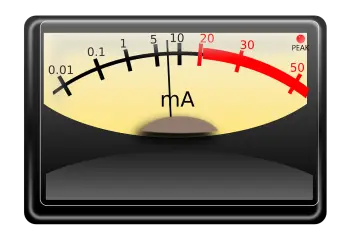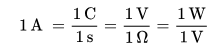AmpereThe ampere (symbol: A) is the SI base unit used to measure the intensity of the electric current.

It takes its name from the French physicist André-Marie Ampère (1775-1836), one of the leading scholars of electromagnetism.

Being one of the seven basic units of the SI, all other electromagnetic units are derived from it. By definition, an ampere is the intensity of an electric current that, if maintained in two parallel linear conductors of infinite length and insignificant cross section, placed one meter away from each other in a vacuum, produces an equal force between them at 2 × 10 -7 N for every meter in length.

The correct way to write ampere is with the initial lowercase letter, except when according to grammar rules a word must be written with the initial use of capital letters.

The ampere is important, therefore, in the field of electricity. This unit appears in the calculation of the sizing of a photovoltaic solar energy installation.

Amp definition

Definition of ampere based on electrical phenomena

The ampere expresses the intensity of the current in a conductor crossed in any section from the charge of a coulomb in the time of a second.

By analogy, the intensity of the current is comparable to the amount of water that passes through a tube measured in kg / second, where the mass of water represents the electrical charge. The latter, that is, the amount of electrons, can be expressed in ampere-hour (Ah), which is the total amount of charge that flows, with the intensity of an amperage, in a conductor in one hour. With such a size, for example, the maximum charge accumulated by the batteries is measured: the car battery contains approximately 55 Ah.

Within the SI units, equality applies:With "C" for coulomb, "s" for second, "V" for volts, "Ω" for ohms and "W" for watts. The absence of coefficients is due to the fact that the International System is a coherent measurement system, which means that the product or the ratio of several units gives rise to a new unit of unit value.

Definition of ampere based on magnetic phenomena

In accordance with the law of Ampere, the ampere can be defined as the current intensity that must flow in two cables of infinite length and is placed at a distance of one meter, so that they attract with a force equal to 2 × 10 - 7   N / m.

Measurement samples

Due to the difficulty in measuring the forces between two conductors, the so-called international ampere or statampere was proposed: defined in terms of the silver deposition rate (the international ampere is the intensity of a current that, through a solution of AgNO 3 [Monovalent Ag], deposited in the cathode 0.001118 g of silver in one second), is equal to 0.99985 A. This unit of measurement, however, is considered obsolete.

Currently, however, most national metrological institutes use sample stacks and resistors for the maintenance of primary amperage samples. The electric current intensity sample is derived from Ohm's law using two samples, one of fem and one of resistance. In Italy, both samples are kept at INRIM in Turin. The fem sample is a group of saturated piles of Weston, controlled by the Josephson effect; The electrical resistance is defined as the average resistance of a group of 10 sample resistors in 1 Ω manganin.

In order to avoid the influence of the contact resistance between the resistance and the measurement circuit, the sample resistors exhibit a particular embodiment four terminals, two external amperometric through which the resistance is subject to the passage of electric current, and two internal voltage compared to the amperometric, of which the voltage drop caused by the circulation of electric current is eliminated.

valoración: 3 - votos 1

Last review: September 7, 2018